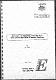## Derivation of a macroscale formulation for a class of nonlinear partial differential equations.1995-05
##### Publisher
Australian Nuclear Science and Technology Organisation
##### Abstract
A macroscale formulation is constructed from a system of partial differential equations which govern the microscale dependent variables. The construction is based upon the requirement that the solutions of the macroscale partial differential equations satisfy in some approximate sense the system of partial differential equations associated with the microscale. These results are restricted to the class of nonlinear partial differential equations which can be expressed as polynomials of the dependent variables and their partial derivatives up to second order. A linear approximation of transformations of second order contact manifolds is employed.
##### Keywords
Differential equations, Fluid flow, Simulation, Porous materials
##### Citation
Pamtelis, G. (1995). Derivation of a macroscale formulation for a class of nonlinear partial differential equations (ANSTO/E722). Lucas Heights, NSW: Australian Atomic Energy Commission.